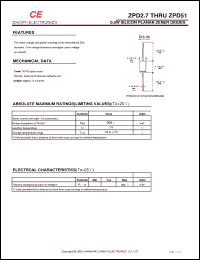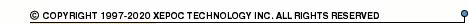More than4 307 195 components listedZPD10 series datasheets. Manufacturer: CNEL.

 ZPD10 0.5W silicon planar zener diode. Zener voltage Vznom = 10 V. Izt = 5 mA. in 2-pin DO-35 package. Operational temperature range from 0°C to 175°C. Datasheet*) ZPD11 0.5W silicon planar zener diode. Zener voltage Vznom = 11 V. Izt = 5 mA. in 2-pin DO-35 package. Operational temperature range from 0°C to 175°C. Datasheet*) ZPD12 0.5W silicon planar zener diode. Zener voltage Vznom = 12 V. Izt = 5 mA. in 2-pin DO-35 package. Operational temperature range from 0°C to 175°C. Datasheet*) ZPD13 0.5W silicon planar zener diode. Zener voltage Vznom = 13 V. Izt = 5 mA. in 2-pin DO-35 package. Operational temperature range from 0°C to 175°C. Datasheet*) ZPD15 0.5W silicon planar zener diode. Zener voltage Vznom = 15 V. Izt = 5 mA. in 2-pin DO-35 package. Operational temperature range from 0°C to 175°C. Datasheet*) ZPD16 0.5W silicon planar zener diode. Zener voltage Vznom = 16 V. Izt = 5 mA. in 2-pin DO-35 package. Operational temperature range from 0°C to 175°C. Datasheet*) ZPD18 0.5W silicon planar zener diode. Zener voltage Vznom = 18 V. Izt = 5 mA. in 2-pin DO-35 package. Operational temperature range from 0°C to 175°C. Datasheet*) ZPD2.7 0.5W silicon planar zener diode. Zener voltage Vznom = 2.7 V. Izt = 5 mA. in 2-pin DO-35 package. Operational temperature range from 0°C to 175°C. Datasheet*) ZPD20 0.5W silicon planar zener diode. Zener voltage Vznom = 20 V. Izt = 5 mA. in 2-pin DO-35 package. Operational temperature range from 0°C to 175°C. Datasheet*) ZPD22 0.5W silicon planar zener diode. Zener voltage Vznom = 22 V. Izt = 5 mA. in 2-pin DO-35 package. Operational temperature range from 0°C to 175°C. Datasheet*) ZPD24 0.5W silicon planar zener diode. Zener voltage Vznom = 24 V. Izt = 5 mA. in 2-pin DO-35 package. Operational temperature range from 0°C to 175°C. Datasheet*) ZPD27 0.5W silicon planar zener diode. Zener voltage Vznom = 27 V. Izt = 5 mA. in 2-pin DO-35 package. Operational temperature range from 0°C to 175°C. Datasheet*) ZPD3 0.5W silicon planar zener diode. Zener voltage Vznom = 3.0 V. Izt = 5 mA. in 2-pin DO-35 package. Operational temperature range from 0°C to 175°C. Datasheet*) ZPD3.3 0.5W silicon planar zener diode. Zener voltage Vznom = 3.3 V. Izt = 5 mA. in 2-pin DO-35 package. Operational temperature range from 0°C to 175°C. Datasheet*) ZPD3.6 0.5W silicon planar zener diode. Zener voltage Vznom = 3.6 V. Izt = 5 mA. in 2-pin DO-35 package. Operational temperature range from 0°C to 175°C. Datasheet*) ZPD3.9 0.5W silicon planar zener diode. Zener voltage Vznom = 3.9 V. Izt = 5 mA. in 2-pin DO-35 package. Operational temperature range from 0°C to 175°C. Datasheet*) ZPD30 0.5W silicon planar zener diode. Zener voltage Vznom = 30 V. Izt = 5 mA. in 2-pin DO-35 package. Operational temperature range from 0°C to 175°C. Datasheet*) ZPD33 0.5W silicon planar zener diode. Zener voltage Vznom = 33 V. Izt = 5 mA. in 2-pin DO-35 package. Operational temperature range from 0°C to 175°C. Datasheet*) ZPD36 0.5W silicon planar zener diode. Zener voltage Vznom = 36 V. Izt = 5 mA. in 2-pin DO-35 package. Operational temperature range from 0°C to 175°C. Datasheet*) ZPD39 0.5W silicon planar zener diode. Zener voltage Vznom = 39 V. Izt = 5 mA. in 2-pin DO-35 package. Operational temperature range from 0°C to 175°C. Datasheet*) ZPD4.3 0.5W silicon planar zener diode. Zener voltage Vznom = 4.3 V. Izt = 5 mA. in 2-pin DO-35 package. Operational temperature range from 0°C to 175°C. Datasheet*) ZPD4.7 0.5W silicon planar zener diode. Zener voltage Vznom = 4.7 V. Izt = 5 mA. in 2-pin DO-35 package. Operational temperature range from 0°C to 175°C. Datasheet*) ZPD43 0.5W silicon planar zener diode. Zener voltage Vznom = 43 V. Izt = 5 mA. in 2-pin DO-35 package. Operational temperature range from 0°C to 175°C. Datasheet*) ZPD47 0.5W silicon planar zener diode. Zener voltage Vznom = 47 V. Izt = 5 mA. in 2-pin DO-35 package. Operational temperature range from 0°C to 175°C. Datasheet*) ZPD5.1 0.5W silicon planar zener diode. Zener voltage Vznom = 5.1 V. Izt = 5 mA. in 2-pin DO-35 package. Operational temperature range from 0°C to 175°C. Datasheet*) ZPD5.6 0.5W silicon planar zener diode. Zener voltage Vznom = 5.6 V. Izt = 5 mA. in 2-pin DO-35 package. Operational temperature range from 0°C to 175°C. Datasheet*) ZPD51 0.5W silicon planar zener diode. Zener voltage Vznom = 51 V. Izt = 5 mA. in 2-pin DO-35 package. Operational temperature range from 0°C to 175°C. Datasheet*) ZPD6.2 0.5W silicon planar zener diode. Zener voltage Vznom = 6.2 V. Izt = 5 mA. in 2-pin DO-35 package. Operational temperature range from 0°C to 175°C. Datasheet*) ZPD6.8 0.5W silicon planar zener diode. Zener voltage Vznom = 6.8 V. Izt = 5 mA. in 2-pin DO-35 package. Operational temperature range from 0°C to 175°C. Datasheet*) ZPD7.5 0.5W silicon planar zener diode. Zener voltage Vznom = 7.5 V. Izt = 5 mA. in 2-pin DO-35 package. Operational temperature range from 0°C to 175°C. Datasheet*) ZPD8.2 0.5W silicon planar zener diode. Zener voltage Vznom = 8.2 V. Izt = 5 mA. in 2-pin DO-35 package. Operational temperature range from 0°C to 175°C. Datasheet*) ZPD9.1 0.5W silicon planar zener diode. Zener voltage Vznom = 9.1 V. Izt = 5 mA. in 2-pin DO-35 package. Operational temperature range from 0°C to 175°C. Datasheet*) There are other manufacturers for this components. For more information please check ZPD10 (BKC) ZPD10 (Diotec Elektronische) ZPD10 (DIODS) ZPD10 (EIC) ZPD10 (FAGOR) ZPD10 (General Semiconductor) ZPD10 (GODAR) ZPD10 (HONEY) ZPD10 (Micronas Intermetall) ZPD10 (Motorola) ZPD10 (Semtech Corp.)